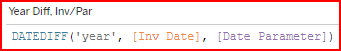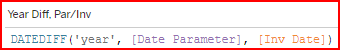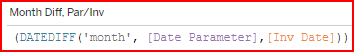# DATEDIFF

DateDiff displays the difference in Years/Months/Days between two date values.
It is useful for selecting multiple time periods in relation to a specified base date.

If you specify 2023 as the base date, the year 2022 has a DateDiff (year) value of -1. It is one year less.
The year 2021 has a DateDiff value of -2, etc. If you create a filter/selection calculation to select when the DateDiff(Year) value is 0, -5, -10, then the years selected will be:
2023-0 = 2023, 2023 -5 = 2017, 2023-10 = 2013.

## YTD calculated Sales measure comparing invoice date to a Date Parameter using the DATEDIFF function

Tableau help link: Creating Year to Date and Month to Date Calculations | Tableau Software

This example shows how to create a YTD SALES calculated field for the year of the Date Parameter using the DATEDIFF function, eliminating the need for a calculated filter. Both methods are useful depending on the situation.

The example above does not account for multiple years, but it can be adapted for that purpose.

Calculating YTD for multiple years gets more complex because you now need to select specific months for each year. This calls for a more complete understanding of how the DATEDIFF function works.
This view, DataSelf DATEDIFF worksheet, shows how the order in which you compare date fields in the formula impacts how the DATEDIFF value is calculated.

Year DateDiff

Month DateDiff[Inv Date] is first, then the [Date Parameter]If [Inv Date] is in the past, it shows a positive number

[Inv Date] is first, then the [Date Parameter]
If [Inv Date] is in the past, it shows a positive number[Parameter Date] is first, then the [Inv Date]If [Inv Date] is in the past, it shows a negative number[Parameter Date] is first, then the [Inv Date]If [Inv Date] is in the past, it shows a negative number

In the formula syntax, the difference is calculated as the 2nd date field minus the 1st date field. In the example, DATEDIFF ('year', [Inv Date], [Date Parameter]), the year of the [Date Parameter] is 2022.
If the Year of the [Inv Date] is 2021, then 2022 - 2021 = 1. The [Date Parameter] year is one greater than the [Inv Date] year. Think of the logic as what needs to be added to the 1st date to get to the 2nd date?

## MTD calculated Sales field as of a Date Parameter using the DATEDIFF function

Tableau help link: Creating Year to Date and Month to Date Calculations | Tableau Software

For MTD Current Year, limit the DATEDIFF ‘month’ value to zero for the current selected month. This eliminates the need for the DATEDIFF ‘year’ line. The day of the month is limited by the [Inv Date] <= [Date Parameter] .

For MTD Prior Year, the DATEDIFF ‘month’ is set to -12.
The day of the month is controlled by the discrete DAY ([Parameter Date]) condition.